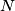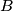« Vrati se

#### MEMO 2010 pojedinačno problem 2

All positive divisors of a positive integer$N$ are written on a blackboard. Two players$A$ and$B$ play the following game taking alternate moves. In the firt move, the player$A$ erases$N$. If the last erased number is$d$, then the next player erases either a divisor of$d$ or a multiple of$d$. The player who cannot make a move loses. Determine all numbers$N$ for which$A$ can win independently of the moves of$B$.